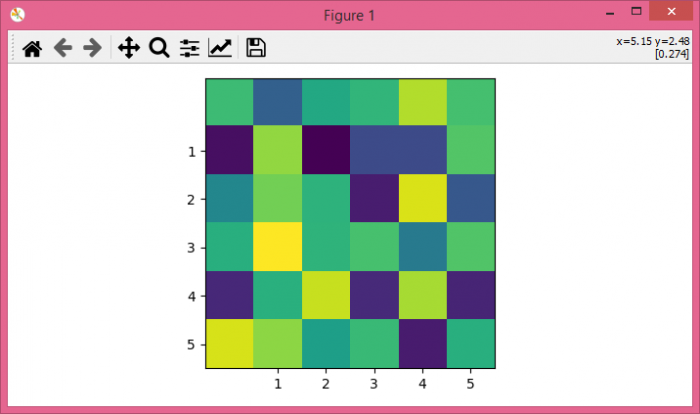# Matplotlib â€“ How to set xticks and yticks with imshow plot?

To set xticks and yticks with imshow() plot, we can take the following steps −

• Set the figure size and adjust the padding between and around the subplots.
• Get the current axis.
• Create a random dataset.
• Display the data as an image, i.e., on a 2D regular raster.
• Set x and y ticks using set_xticks() and set_yticks() method.
• To display the figure, use show() method.

## Example

import numpy as np
import matplotlib.pyplot as plt

plt.rcParams["figure.figsize"] = [7.00, 3.50]
plt.rcParams["figure.autolayout"] = True

ax = plt.gca()
data = np.random.rand(6, 6)
ax.imshow(data)

# Set xticks and yticks
ax.set_xticks([1, 2, 3, 4, 5])
ax.set_yticks([1, 2, 3, 4, 5])

plt.show()

## Output

It will produce the following output